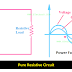Hey, today we are going to know what will be the power factor of an alternating circuit containing 1 pure resistor? It is very easy to understand. Just start thinking from basics. First of all, let's go to know what is the power factor.

When an alternating current flowing in an electrical circuit, there is some phase difference occurs between voltage and current. This phase difference depends, what type of load is connected in that circuit. The power factor indicates that phase difference.

So the power factor is nothing but the cosine of the angle between voltage and current.

Suppose an alternating circuit contains only one pure resistor that means it is a purely resistive circuit. In a purely resistive circuit current always flows in the same phase with the voltage, it does not matter what type of supply(AC or DC) applied to that circuit because of resistor shows the same characteristics for both AC and DC supply.

Here you can see in the below figure, that the electrical circuit contains only one resistor.

You can also see the voltage and current both are in the same phase.

So, it is clear that as the current flows in the same phase with the voltage for the purely resistive circuit, there is no phase difference that means the angle between voltage and current is zero or θ = 0

So, the power factor will be, cosθ = cos0 = 1

The power factor of an alternating circuit will be one or Unity if it containing 1 pure resistor.

Thank you for visiting the website. keep visiting for more updates.

Power factor of alternating circuit containing pure ResistorReviewed by manoranjan das on 2/21/2020 Rating: 5